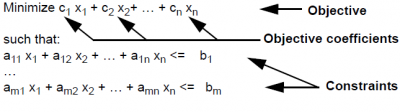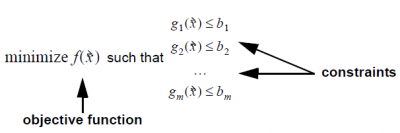# Optimization Characteristics

This page introduces optimization and the different types of optimization problem. It is not specific to Analytica Optimizer, and applies to any kind of optimization or solver software. It explains:

• The basic elements of an optimization, including decision variables, objective, and constraints.
• The different types of optimization problems, linear programs (LP), quadratic programs (QP), and (other) nonlinear programs (NLP).
• Identifying continuous, discrete, or mixed variables
• Solving simultaneous equations and other constrain satisfaction problems

## Parts of an Optimization Problem

The first step in performing an optimization is to formulate the problem appropriately. An optimization problem is defined by four parts: a set of decision variables, an objective function, bounds on the decision variables, and constraints. The formulation looks like this.### Decision variables

A vector (one-dimensional array) of the decision variables whose values we can change to find an optimal solution $\displaystyle{ \vec x }$ = (x1, x2, …, xn) n. A solution is a set of values assigned to these decision variables.

### Objective

The Objective is a function, $\displaystyle{ f(\vec x) }$, of the decision variables. It gives a single number evaluating a solution, which the Optimizer tries to minimize or maximize, whichever you specify in the formulation. For a linear program (LP), the objective is defined by a set of coefficients or weights that apply to the decision variables. For a nonlinear program (NLP), the objective can be any expression or variable that depends on the decision variables.

### Bounds

A range lbi ≤ xi ≤ ubi, i = 1..n on the decision variables, defining what values are allowed. These bounds define the set of possible solutions, called the search space. Each decision variable can have a lower bound and/or an upper bound. If not specified, the lower and upper bounds are -INF and +INF — that is, there are no bounds.

### Constraints

The constraints, e.g. g1($\displaystyle{ \vec x }$) ≤ b1, are bounds on functions of the decision variables. They define which solutions are feasible.

## Types of optimization

An optimization problem may be linear, quadratic, or (other) nonlinear. Analytica can automatically determine the type from analyzing the model, and choose the most suitable solver engine from those it has available. But, you will find it helpful to have a basic understanding of what these classes mean.

For a linear program (LP), the objective must be a linear function of the decision variables. For a quadratic program (QP), the objective is a quadratic function and the constraints must be linear functions of the decision variables. For a quadratically-constrained program (QCP), the objective and constraints must all be linear or quadratic functions of decision variables. The problem is a nonlinear program (NLP) if the objective or any of the constraints are non-quadratic in any of the decision variables.

Linear and convex quadratic optimization problems are often relatively fast to compute. But general nonlinear optimization is a computationally difficult problem. Many of the most famous and notoriously difficult computation problems can be cast as optimization programs, from the traveling salesman to the solution (or non-solution) of Fermat’s last “theorem.” It is, therefore, unreasonable to expect the Optimizer engine to succeed on any possible nonlinear problem you can formulate. While the Frontline Solver engine used in the Analytica Optimizer is one of the better general-purpose optimization engines available, success with hard optimization problems depends on your ability to formulate the problem effectively, provide appropriate hints for the Optimizer, and adjust the search control settings.

There are often several ways to formulate the same problem. Since linear and quadratic formulations are usually so much faster and easier to solve than nonlinear problems, it is worth careful thought to see if it is possible to reformulate a nonlinear problem so that it is linear or quadratic. You can often achieve that with a simple transformation, combination, or disaggregation of the decision variables.

## Specific Optimization Characteristics

The general description of optimization problems applies to all problem types. This section provides more specific mathematical descriptions for each type of optimization.

### Parts of a Linear Program (LP)

A linear optimization problem has the following standard formulation.In this standard form, all decision variables, xi, are real-valued and unconstrained, ranging from -INF to +INF (-∞ to ∞).

Linear programs generally solve quickly, although large models can be computationally complex. Solutions to linear problems always represent a global maximum or minimum. This means that you always be assured of finding the absolute optimum solution.

### Parts of a Quadratic Program (QP)

A quadratic program has the following standard formulation:The objective and constraint left-hand sides are written here in matrix notation. The constraints are the same as they were in the LP formulation, and differ here only by the fact that they appear here in matrix notation. The term $\displaystyle{ \vec c }$ · $\displaystyle{ \vec x }$ is the linear part of the objective, and the $\displaystyle{ \vec x }$T$\displaystyle{ \varrho \vec x }$ is the quadratic part of the objective,which is specified by the $\displaystyle{ \, n \times \, n }$ matrix $\displaystyle{ \varrho \times \vec x }$T denotes the vector transpose of the decision variables.

### Parts of a Quadratically-Constrained Program (QCP)This formulation augments the pure quadratic program by adding quadratic terms to the constraints. It may be the case that most constraints are linear, so that the $\displaystyle{ \varrho }$i matrices for those constraints are not present (or 0), but if even a single constraint is quadratic, the problem is classified as a QCP.

The quadratic terms, i.e. $\displaystyle{ \vec x }$T$\displaystyle{ \varrho \vec x }$, in the objective and $\displaystyle{ \vec x }$T$\displaystyle{ \varrho }$i$\displaystyle{ \vec x }$in the constraint are specified by the n x n matrices , where is the number of decision variables and is the number of constraints, and denotes the vector transpose of the decision variables.

### Parts of a Non-Linear Program (NLP)

The basic formulation for a nonlinear optimization iswhere is a vector denoting the n-dimensional candidate solution.

A nonlinear program (NLP) is the most general formulation for an optimization. The objective and the constraints can be arbitrary functions of the decision variables, continuous or discontinuous. This generality comes at the price of longer computation times, and less precision than linear and quadratic programs (LP and QP). There is also the possibility with smooth NLPs that the Optimizer will return a local optimum that is not the global optimum solution. In general, it is hard to prove whether a solution is globally optimal or not. For these reasons, it is better to reformulate nonlinear problems as linear or quadratic when possible.

When a model is linear, quadratic, or quadratically constrained, DefineOptimization() analyzes the model and all the definitions contained within, and determines the coefficients for the objective and each constraint. Solver engines are able to apply special algorithms to these coefficient matrices, and avoid repeated evaluations and finite differencing of your model. However, once your problem is non-linear, the solver engines must repeatedly re-evaluate your model, often multiple times around a single search point in order to estimate gradients. All the engine can infer about the search space are the results of these evaluations. Hence, a solver of a non-linear problem has less information to work with, and thus has a much more difficult task to perform.

## Continuous, integer, and mixed-integer programs

Each decision variable can be specified as continuous, meaning it is a real number (between bounds if specified), as semi-continuous (a real number with bounds, or zero), as integer, meaning a whole number, as binary or Boolean, meaning its values can be True (1) or False (0), as a member of an integer group, where each member of the group must have a different integer value, or as one of a finite set of explicit discrete categorical labels. Optimization problems are classified as continuous, meaning the decision variables are all continuous; integer, meaning they are all integer, binary, or group variables; or mixed-integer if they are a mixture of continuous, semi-continuous, integer, binary, group, or discrete variables. In this naming convention, binary or Boolean variables are treated as integer variables. The Optimizer engine uses these distinctions to select which algorithms to use.

## Solving simultaneous equations

The Optimizer first attempts to find a feasible solution. If found, it then attempts to optimize within the set of feasible solutions. Thus, solving a set of simultaneous equations is a special case of the optimization problem, where each constraint has a sense of =, the objective is irrelevant (unless you want to express a preference among feasible solutions), and any feasible solution is a solution to the system of equations.